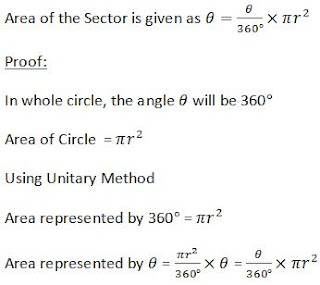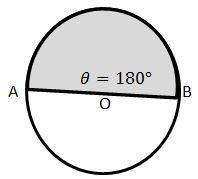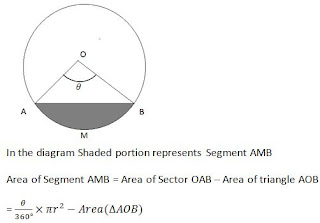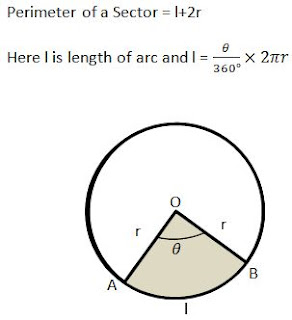>

## Revision Notes for Ch 12 Areas related to Circles Class 10th Mathematics

Area of Sector

Sector: Sector of a Circle is given as part of Circle enclosed by 2 radius and arc.In the diagram, shaded area OAB is the sector.
Here, θ is the angle subtended by the arc AB on the center O of the circle.Area of Semi CircleSemi-Circle is a sector forming an angle 180° with center.
θ=180°
Now, Area of Semi Circle will be given asArea of Quarter CircleArea of Segment

Segment is defined as area enclosed by chord and arc of the circle.Perimeter of Sector

• It is the length which enclosed the sector.

• Length of arc AB + OA + OB.

• OA=OB=r, that is radius of sector.

So,